Home
Hostname: page-component-684899dbb8-gblv7 Total loading time: 0.171 Render date: 2022-05-29T12:39:18.742Z Has data issue: true Feature Flags: { "shouldUseShareProductTool": true, "shouldUseHypothesis": true, "isUnsiloEnabled": true, "useRatesEcommerce": false, "useNewApi": true }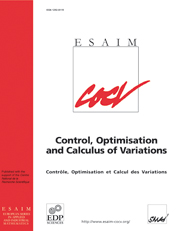ESAIM: Control, Optimisation and Calculus of Variations

# The regularisation of the N-wellproblem by finite elements and by singular perturbation are scalingequivalent in two dimensions

Published online by Cambridge University Press:  24 June 2008

## Abstract

Let $K:=SO\left(2\right)A_1\cup SO\left(2\right)A_2\dots SO\left(2\right)A_{N}$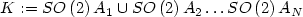where $A_1,A_2,\dots, A_{N}$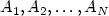are matrices of non-zero determinant. We establish a sharp relation between the following two minimisation problems in two dimensions. Firstly the N-well problem with surface energy. Let $p\in\left[1,2\right]$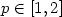, $\Omega\subset \mathbb{R}^2$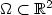be a convex polytopal region. Define $$I^p_{\epsilon}\left(u\right)=\int_{\Omega} d^p\left(Du\left(z\right),K\right)+\epsilon\left|D^2 u\left(z\right)\right|^2 {\rm d}L^2 z$$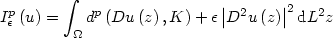and let AF denote the subspace of functions in $W^{2,2}\left(\Omega\right)$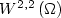that satisfy the affine boundary condition Du=F on $\partial \Omega$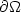(in the sense of trace), where $F\not\in K$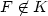. We consider the scaling (with respect to ϵ) of $$m^p_{\epsilon}:=\inf_{u\in A_F} I^p_{\epsilon}\left(u\right).$$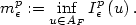Secondly the finite element approximation to the N-well problem without surface energy. We will show there exists a space of functions $\mathcal{D}_F^{h}$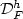where each function $v\in \mathcal{D}_F^{h}$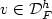is piecewise affine on a regular (non-degenerate) h-triangulation and satisfies the affine boundary condition v=lF on $\partial \Omega$(where lF is affine with $Dl_F=F$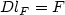) such that for $$\alpha_p\left(h\right):=\inf_{v\in \mathcal{D}_F^{h}} \int_{\Omega}d^p\left(Dv\left(z\right),K\right) {\rm d}L^2 z$$there exists positive constants $\mathcal{C}_1<1<\mathcal{C}_2$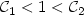(depending on $A_1,\dots, A_{N}$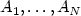, p) for which the following holds true $$\mathcal{C}_1\alpha_p\left(\sqrt{\epsilon}\right)\leq m^p_{\epsilon}\leq \mathcal{C}_2\alpha_p\left(\sqrt{\epsilon}\right) \text{ for all }\epsilon>0.$$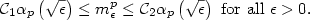Type
Research Article
Information

## Access options

Get access to the full version of this content by using one of the access options below. (Log in options will check for institutional or personal access. Content may require purchase if you do not have access.)

## References

Ambrosio, L., Coscia, A. and Dal Maso, G., Fine properties of functions with bounded deformation. Arch. Rational Mech. Anal. 139 (1997) 201238. CrossRef
L. Ambrosio, N. Fusco and D. Pallara, Functions of bounded variation and free discontinuity problems, Oxford Mathematical Monographs. The Clarendon Press, Oxford University Press, New York (2000).
Ball, J.M. and James, R.D., Fine phase mixtures as minimisers of energy. Arch. Rational Mech. Anal. 100 (1987) 1352. CrossRef
Ball, J.M. and James, R.D., Proposed experimental tests of a theory of fine microstructure and the two well problem. Phil. Trans. Roy. Soc. London Ser. A 338 (1992) 389450. CrossRef
Chipot, M., The appearance of microstructures in problems with incompatible wells and their numerical approach. Numer. Math. 83 (1999) 325352. CrossRef
Chipot, M. and Kinderlehrer, D., Equilibrium configurations of crystals. Arch. Rational Mech. Anal. 103 (1988) 237277.
M. Chipot and S. Müller, Sharp energy estimates for finite element approximations of non-convex problems, in Variations of domain and free-boundary problems in solid mechanics (Paris, 1997), Solid Mech. Appl. 66, Kluwer Acad. Publ., Dordrecht (1999) 317–325.
Conti, S., Branched microstructures: scaling and asymptotic self-similarity. Comm. Pure Appl. Math. 53 (2000) 14481474. 3.0.CO;2-C>CrossRef
Conti, S. and Schweizer, B., Rigidity and Gamma convergence for solid-solid phase transitions with $SO(2)$-invariance. Comm. Pure Appl. Math. 59 (2006) 830868. CrossRef
Conti, S., Faraco, D. and Maggi, F., A new approach to counterexamples to $L\sp 1$estimates: Korn's inequality, geometric rigidity, and regularity for gradients of separately convex functions. Arch. Rational Mech. Anal. 175 (2005) 287300. CrossRef
Conti, S., Dolzmann, G. and Kirchheim, B., Existence of Lipschitz minimizers for the three-well problem in solid-solid phase transitions. Ann. Inst. H. Poincaré Anal. Non Linéaire 24 (2007) 953962. CrossRef
Dacorogna, B. and Marcellini, P., General existence theorems for Hamilton-Jacobi equations in the scalar and vectorial cases. Acta Math. 178 (1997) 137. CrossRef
C. De Lellis and L. Székelyhidi, The Euler equations as a differential inclusion. Ann. Math. (to appear).
Dolzmann, G. and Bhattacharya, K., Relaxed constitutive relations for phase transforming materials. The J. R. Willis 60th anniversary volume. J. Mech. Phys. Solids 48 (2000) 14931517.
Dolzmann, G. and Kirchheim, B., Liquid-like behavior of shape memory alloys. C. R. Math. Acad. Sci. Paris 336 (2003) 441446. CrossRef
Dolzmann, G. and Müller, S., Microstructures with finite surface energy: the two-well problem. Arch. Rational Mech. Anal. 132 (1995) 101141. CrossRef
L.C. Evans and R.F. Gariepy, Measure theory and fine properties of functions, Studies in Advanced Mathematics. CRC Press, Boca Raton, FL (1992).
Friesecke, G., James, R.D. and Müller, S., A theorem on geometric rigidity and the derivation of nonlinear plate theory from three dimensional elasticity. Comm. Pure Appl. Math. 55 (2002) 14611506. CrossRef
Kirchheim, B., Deformations with finitely many gradients and stability of quasiconvex hulls. C. R. Acad. Sci. Paris Sér. I Math. 332 (2001) 289294. CrossRef
B. Kirchheim, Rigidity and Geometry of Microstructures. Lectures note 16/2003, Max Planck Institute for Mathematics in the Sciences, Leipzig (2003).
R.V. Kohn, New Estimates for Deformations in Terms of Their Strains. Ph.D. thesis, Princeton University, USA (1979).
Kohn, R.V. and Müller, S., Surface energy and microstructure in coherent phase transitions. Comm. Pure Appl. Math. 47 (1994) 405435. CrossRef
Lorent, A., An optimal scaling law for finite element approximations of a variational problem with non-trivial microstructure. ESAIM: M2AN 35 (2001) 921934. CrossRef
Luskin, M., On the computation of crystalline microstructure. Acta Numer. 5 (1996) 191257. CrossRef
P. Mattila, Geometry of Sets and Measures in Euclidean Spaces, Cambridge Studies in Advanced Mathematics 44. Cambridge University Press (1995).
Müller, S., Singular perturbations as a selection criterion for periodic minimizing sequences. Calc. Var. Partial Differ. Equ. 1 (1993) 169204. CrossRef
S. Müller, Variational models for microstructure and phase transitions, in Calculus of variations and geometric evolution problems (Cetraro, 1996), Lecture Notes in Mathematics 1713, Springer, Berlin (1999) 85–210. www.mis.mpg.de/cgi-bin/lecturenotes.pl.
S. Müller, Uniform Lipschitz estimates for extremals of singularly perturbed nonconvex functionals. MIS MPG, Preprint 2 (1999).
S. Müller and V. Šverák, Attainment results for the two-well problem by convex integration, in Geometric Analysis and the Calculus of Variations. For Stefan Hildebrandt, J. Jost Ed., International Press, Cambridge (1996) 239–251.
Müller, S. and Šverák, V., Convex integration with constraints and applications to phase transitions and partial differential equations. J. Eur. Math. Soc. (JEMS) 1 (1999) 393422.
Müller, S. and Šverák, V., Convex integration for Lipschitz mappings and counterexamples to regularity. Ann. Math. 157 (2003) 715742. CrossRef
Müller, S., Rieger, M. and Šverák, V., Parabolic systems with nowhere smooth solutions. Arch. Rational Mech. Anal. 177 (2005) 120. CrossRef
Sychev, M.A., Comparing two methods of resolving homogeneous differential inclusions. Calc. Var. Partial Differ. Equ. 13 (2001) 213229. CrossRef
Sychev, M.A. and Müller, S., Optimal existence theorems for nonhomogeneous differential inclusions. J. Funct. Anal. 181 (2001) 447475.
V. Šverák, On the problem of two wells, in Microstructure and phase transition, D. Kinderlehrer, R.D. James, M. Luskin and J. Ericksen Eds., IMA Vol. Math. Appl. 54, Springer, New York (1993) 183–189.

# Save article to Kindle

Note you can select to save to either the @free.kindle.com or @kindle.com variations. ‘@free.kindle.com’ emails are free but can only be saved to your device when it is connected to wi-fi. ‘@kindle.com’ emails can be delivered even when you are not connected to wi-fi, but note that service fees apply.

Find out more about the Kindle Personal Document Service.

The regularisation of the N-well problem by finite elements and by singular perturbation are scaling equivalent in two dimensions
Available formats
×

# Save article to Dropbox

To save this article to your Dropbox account, please select one or more formats and confirm that you agree to abide by our usage policies. If this is the first time you used this feature, you will be asked to authorise Cambridge Core to connect with your Dropbox account. Find out more about saving content to Dropbox.

The regularisation of the N-well problem by finite elements and by singular perturbation are scaling equivalent in two dimensions
Available formats
×

# Save article to Google Drive

To save this article to your Google Drive account, please select one or more formats and confirm that you agree to abide by our usage policies. If this is the first time you used this feature, you will be asked to authorise Cambridge Core to connect with your Google Drive account. Find out more about saving content to Google Drive.

The regularisation of the N-well problem by finite elements and by singular perturbation are scaling equivalent in two dimensions
Available formats
×
×